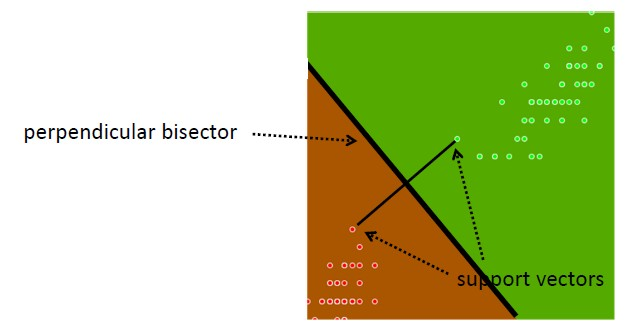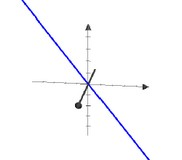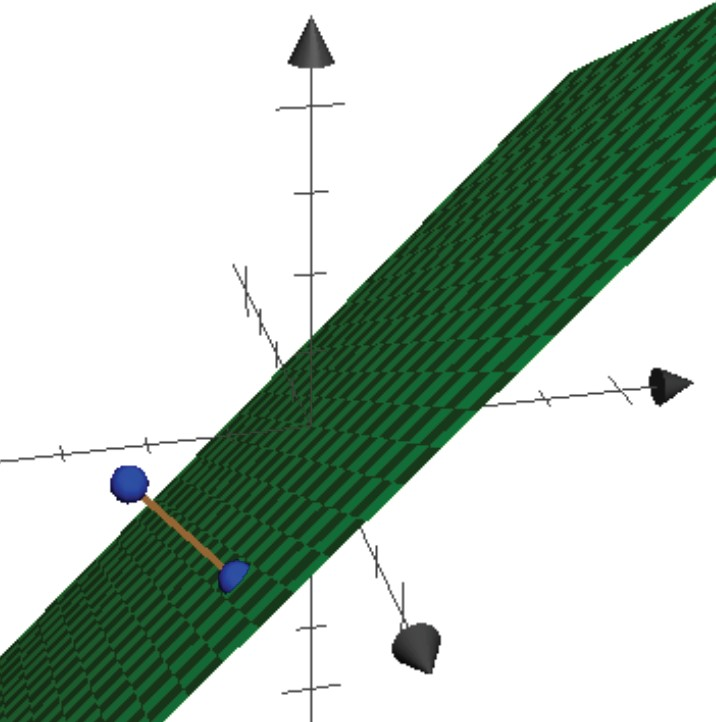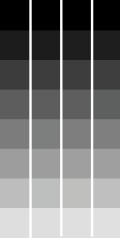# Linear Algebra - Plane

A plane is a two dimensional vector space.

A plane has a dimension of two because two coordinates are needed to specify a point on it.

## Type

### Containing the origin

• Two-dimensional: All points in the plane: Span {[1, 2], [3, 4]}
• Span of two 3-vectors {[1, 0, 1.65], [0, 1, 1]} is a plane in three dimensions.
# A more familiar way to specify a plane
{(x, y, z) : ax + by + cz = 0}
# Using dot-product, the above equation becomes a set of vectors
# satisfying a linear equation with right-hand side zero
{[x, y, z] : [a, b, c] * [x, y, z] = 0}


#### Plane Intersection

The intersection of the two following plane:

• {[x, y, z] : [4,-1, 1] · [x, y, z] = 0}
• {[x, y, z] : [0, 1, 1] · [x, y, z] = 0}

is

• {[x, y, z] : [4,-1, 1] · [x, y, z] = 0, [0, 1, 1] · [x, y, z] = 0}

### Translation

The translation of a plane translate it in a way that it doesn't contain the origin.

You can express such plane as

• an affine hull
• a solution set of an equation

Vector addition is used to defined a set of points forming an plane that not necessarily go through the origin.

You translate the plane by adding a vector c [0.5, 1] to every point in the plane.abbreviated: $c + nu$

The result is a plane through c instead of through origin.

#### Equation

The solution set of an linear equation:

• ax + by + cz = d
• In vector terms:{[x, y, z] : [a, b, c] · [x, y, z] = d}

Discover MoreData Mining - Support Vector Machines (SVM) algorithm

A support vector machine is a Classification method. supervised algorithm used for: Classification and Regression (binary and multi-class problem) anomalie detection (one class problem) Supports:...Geometry - Object

in Geometry An object can be: a pre-defined shape or an arbitrary one defined with the help of a path. Notions such as: The length of a vector The angle between two vectors. are introduced...Geometry - Shape (with transformation and notion)

in Geometry A shape is any list of line drawing commands, which strung together, describe a continuous line or closed shape that can be filled or stroked. Move, Line, Quadratic and Cubic...Linear Algebra - Linear combination

A linear combination of the vectors is the following expression: where: The scalars are the coefficients Every vector in is a linear combination: Every linear combination is a vector in...Linear Algebra - (Direct Sum | Union) of vector spaces

Let U and V be two vector spaces consisting of D-vectors over a field F. Definition: If U and V share only the zero vector then we define the direct sum of U and V to be the set: written: That is, ...Linear Algebra - Closest point in a plane

Given a point b and a plane, find the point in the plane closest to b. By , we can assume the plane includes the origin. The plane is a vector space V. Let {v1, v2} be a basis for V. Goal: Given...Linear Algebra - Point

Zero-dimensional: Span of the empty set: The origin A point (x, y) can be represented in the plane by a {'x','y'}-vector. A point can also be represented by {'x','y','z'}-vector. This representation...Linear Algebra - Span of a Vector Space

The set of all linear combinations of some vectors v1,...,vn is called the span of these vectors and contains always the origin. Example: Let V = Span {[0, 0, 1], [2, 0, 1], [4, 1, 2]}. A vector belongs...Linear Algebra - Vector

tuple in Linear algebra are called vector. A vector is a list of scalar (real number) used to represent a When the letters are in bold in a formula, it signifies that they're vectors, To represent...Linear Algebra - Vector Space (set of vector)

A vector space is a subset of the set of function representing a geometric objectpassing through the origin. A vector space over a field F is any set V of vector : with the addition and scalar-multiplication...